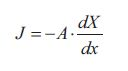Chemistry

# What is Transport Phenomena?

Things only move when they are forced to move! A bicycle only moves when a force is applied in the form of pedaling. A cloud on the sky only moves when a force is applied in the form of a storm or a wind. A soccer ball only flies into the goal net when a force in the form of a beautiful and precise shot from Peter Moller or another great soccer player is applied. Thus all sorts of transport only take place when a force, called a driving force, is applied. This is also the case when we are talking about transport of other things than bicycles, clouds and soccer balls.

Transport of mass, energy, momentum, volume and electricity only takes place when a driving force is applied. Transport can generally be expressed as a flux “ʃ”, which is given by the amount of mass, energy, momentum, volume or charges that are transported pr. area pr. time. The transported amount is proportional to the applied driving force, which can be expressed by a linear phenomenological equation of the following kind:The transport direction in this case is in the x-direction. In this text we are only dealing with the one-dimensional case. Analogies to two- and three dimensional cases can be found in teaching books about transport phenomena. The driving force is expressed as the gradient of X (concentration, temperature, velocity, pressure or voltage) along an x-axis parallel to the transport direction. Since transport always goes “downhill” from areas of high concentration, temperature, velocity etc. to areas of low concentration, temperature, velocity etc., a minus-sign is placed on the right side of the equation because the flux should be positive when the gradient dX/dx is negative. The proportionality constant A is called a phenomenological coefficient and is related to many well known physical terms associated with different kinds of transport. Table I lists different kinds transport together with the driving forces, phenomenological flux equations, names of the phenomenological coefficients, units of the different fluxes and the common name for the transport phenomena.